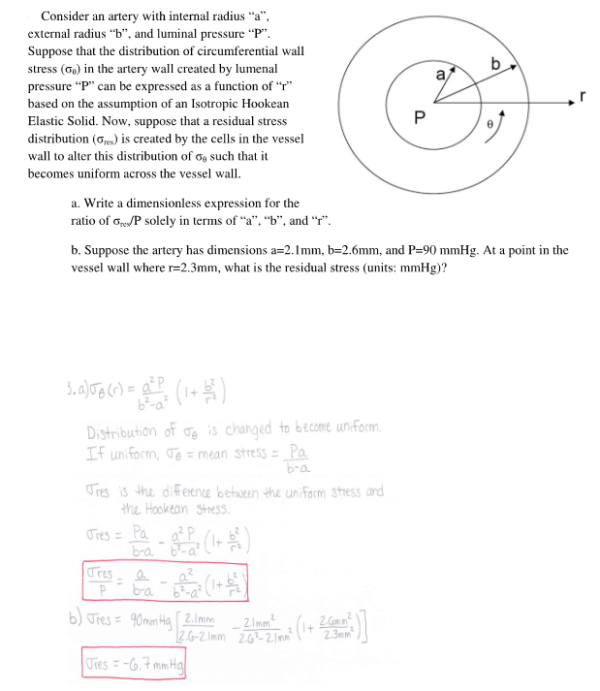Consider an artery with internal radius a, external radius b, and luminal pressure P. Suppose that the distribution of circumferential wall stress in the artery wall created by lumenal pressure P can be expressed as a function of r based on the assumption of an Isotropic Hookean Elastic Solid. Now, suppose that a residual stress distribution is created by the cells in the vessel wall to alter this distribution of sigma such that it becomes uniform across teh vessel wall. a) Write a dimensionless expresson for the ratio of sigma/P solely in terms of a, b and r. b) Suppose the artery has dimensions a=2.1 mm, b=2.6 mm, and P=90 mmHg. At a point in the vessel wall where r=2.3 mm, what is the residual stress?Consider an artery with internal radius a, external radius b, and luminal pressure P. Suppose that the distribution of circumferential wall stress in the artery wall created by lumenal pressure P can be expressed as a function of r based on the assumption of an Isotropic Hookean Elastic Solid. Now, suppose that a residual stress distribution is created by the cells in the vessel wall to alter this distribution of sigma such that it becomes uniform across teh vessel wall. a) Write a dimensionless expresson for the ratio of sigma/P solely in terms of a, b and r. b) Suppose the artery has dimensions a=2.1 mm, b=2.6 mm, and P=90 mmHg. At a point in the vessel wall where r=2.3 mm, what is the residual stress?

biomechanics page 1 biomechanics biomechanics biomechanics biomechanics biomechanics biomechanics biomechanics biomechanics page 2